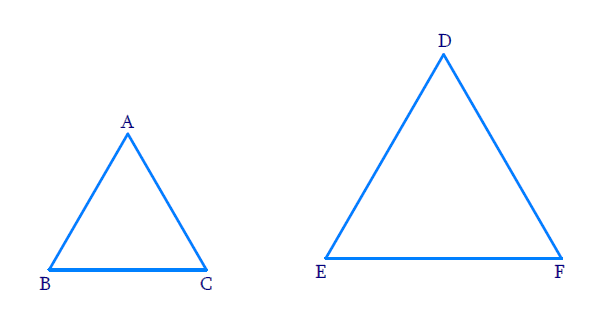# Ex.6.4 Q4 Triangles Solution - NCERT Maths Class 10

Go back to  'Ex.6.4'

## Question

If the areas of two similar triangles are equal, prove that they are congruent.

DiagramVideo Solution
Triangles
Ex 6.4 | Question 4

## Text Solution

Reasoning:

As we know that two triangles are similar if their corresponding angles are equal and their corresponding sides are in the same ratio.

The ratio of the areas of two similar trianglesis equal to the square of the ratio of their corresponding sides.

As we know if three sides oh one triangle are equal to the three sides of another triangle,then the two triangles are congruent.

Steps:

$$\Delta ABC \sim \Delta DEF$$

\begin{align}\Rightarrow \quad&\frac{{AB}}{{DE}} = \frac{{BC}}{{EF}} = \frac{{CA}}{{FD}}\\ & \text{(SSS Criterion)} \end{align}

\begin{align}&\;ar\Delta ABC=\Delta DEF\\\Rightarrow\quad&\frac{{ar\Delta ABC}}{{ ar\Delta DEF}} = 1\;\;\dots(1)\end{align}

But,

\begin{align}\frac{{ar\Delta ABC}}{{ar\Delta DEF}} &= \frac{{{{(AB)}^2}}}{{{{(DE)}^2}}} \\= \frac{{{{(BC)}^2}}}{{{{(EF)}^2}}} &= \frac{{{{(CA)}^2}}}{{{{(FD)}^2}}}\end{align}

From $$(1)$$

\begin{align}{\frac{(A B)^{2}}{(D E)^{2}}}&=\frac{(B C)^2}{(E F)^2} \\ & =\frac{(C A)^2}{(F D)^2} \\ & =1 \\ {\Rightarrow \; \frac{(A B)^{2}}{(D E)^{2}}}&={1} \\ {\Rightarrow \;\; (AB)^{2}}&={(D E)^{2} }\\ \Rightarrow \;\;\quad A B &=DE \;\;\ldots (2)\end{align}

Similarly,

$$\Rightarrow$$   $$BC = EF .... (3)$$

$$\Rightarrow$$   $$CA =FD …. (4)$$

Now, in $$\Delta ABC,\,\Delta DEF$$

$$\Rightarrow$$$$AB = DE$$    $$\text{(from 2)}$$

$$\Rightarrow$$$$BC = EF$$    $$\text{(from 3)}$$

$$\Rightarrow$$$$CA = FD$$    $$\text{(from 4)}$$

\begin{align} \Rightarrow\quad &\Delta ABC \cong \Delta DEF\\& \text{(SSS congruency)}\end{align}

Learn from the best math teachers and top your exams

• Live one on one classroom and doubt clearing
• Practice worksheets in and after class for conceptual clarity
• Personalized curriculum to keep up with school